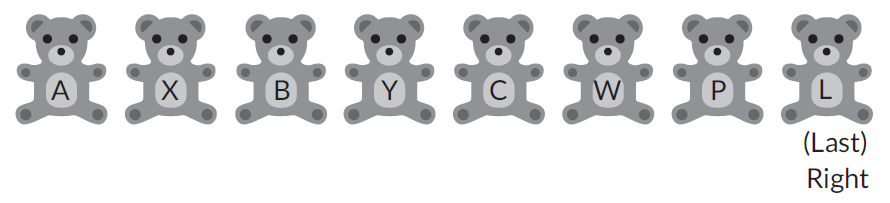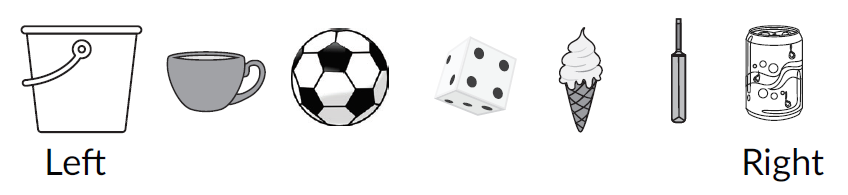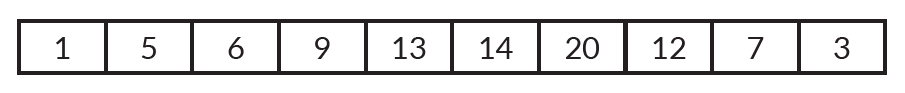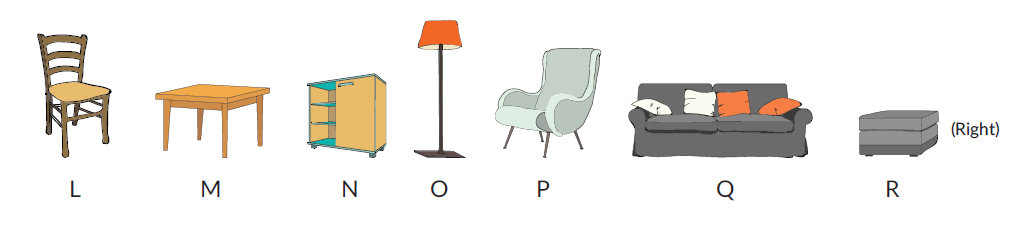Math & Beyond

# What is Ranking? How do we rank objects?

128 views

 1 What is Ranking? 2 How does ranking help in practical life? 3 Standard Competition Ranking (SCR) 4 Ordinal Ranking 5 Fractional Ranking 6 How To Solve Ranking Problems? 7 Solved Examples 8 Conclusion 9 Frequently Asked Questions (FAQs) 10 External References

22 December 2020

Hello readers, in this blog we will discuss the topic ‘Ranking of an object’ or position of a person from left to right or top to bottom alignment. We shall also go through some examples.
So let’s get started!

## What is Ranking?

Ranking is the arrangement of objects according to their position, or in the given order from left to right or top to bottom. It means that you give each object a rank or position with respect to a reference point.

For example, a person is standing in a line. His position is 5th from the right.

## What is Ranking? How do we rank objects?

A ranking is a relationship between a set of items such that, for any two items, the first is either "ranked higher than", "ranked lower than" or "ranked equal to" the second. In mathematics, this is known as a weak order or total preorder of objects.

What is Ranking? How do we rank objects? Is mentioned below in the Downloadable PDF.

## How does ranking help in practical life?

Ranking helps us to locate a particular object/person from a group of them placed in a particular order.

Ranking is particularly useful in analyzing statistics where it is used to organize and identify data. Apart from ranking in statistics, it is useful for comparison of the positions of two or more different things or persons.

It helps in arranging things or people in a particular order, and also helps in locating the position of the person standing in the queue.

Practical examples where ranking is used are:

• Comparing objects or people
• The rank of a matrix
• Locating a particular thing or person, etc.

There are many types of official rankings, and often each tournament will have its own rules and regulations regarding the ranking system.

•  Standard competition ranking
•  Ordinal ranking
•  Fractional ranking.
1. ### Standard Competition Ranking (SCR)

A ranking system in which the mathematical values that are equal are assigned an equal rank, and the next, lesser value is given the next highest rank.

1. ### Ordinal Ranking

A system of ordering where each mathematical value is given a particular position in a sequence of numbers where no positions are equal.

1. ### Fractional Ranking

The is a system of order in which the mathematical values that are equal are given the mean of the ranking positions.

## How To Solve Ranking Problems?

Here, we have provided steps to solve problems based on ranking:

Steps to Solve:
Step 1: See carefully all items given in the figure.
Step 2: Identify if the question asks ordering from top to bottom or left to right.
Step 3: According to the question mark the top and bottom or left and right.
Step 4: Identify the position of an object/ a person from the left end or right end or from the top or bottom.
Step 5: Think and choose the right option.

## Solved Examples

Example: Which teddy bear is to the immediate right of the third teddy bear?(A) X

(B) Y

(C) W

(D) C

 (B) Y

Example: If there was no ice-cream, what would be the position of the cup?(A) 2nd from the right
(B) 5th from the right
(C) 5th from the left
(D) 4th from the left

 (B) 5th from the right

Example: Which number is eighth from the right?(A) 6

(B) 13

(C) 9

(D) 12

 (A) 6

Examples: If items O and P are removed from the given arrangement then the fourth item from the left end is _____.(A) O

(B) Q

(C) N

(D) R

 (B) Q

## Conclusion

In mathematics, ranking is known as a weak order or total preorder of objects. It is not necessarily a total order of objects because two different objects can have the same ranking. The rankings themselves are totally ordered.

Therefore, ranking is useful in various fields, like job evaluation, in the exam system, analyzing, and ordering of the objects, etc.

Cuemath, a student-friendly mathematics and coding platform, conducts regular Online Live Classes for academics and skill-development, and their Mental Math App, on both iOS and Android, is a one-stop solution for kids to develop multiple skills. Understand the Cuemath Fee structure and sign up for a free trial.

## What is Ranking?

Ranking is the arrangement of objects according to their position, or in the given order from left to right or top to bottom. It means that you give each object a rank or position with respect to a reference point.

## External References

Related Articles
GIVE YOUR CHILD THE CUEMATH EDGE
Access Personalised Math learning through interactive worksheets, gamified concepts and grade-wise courses